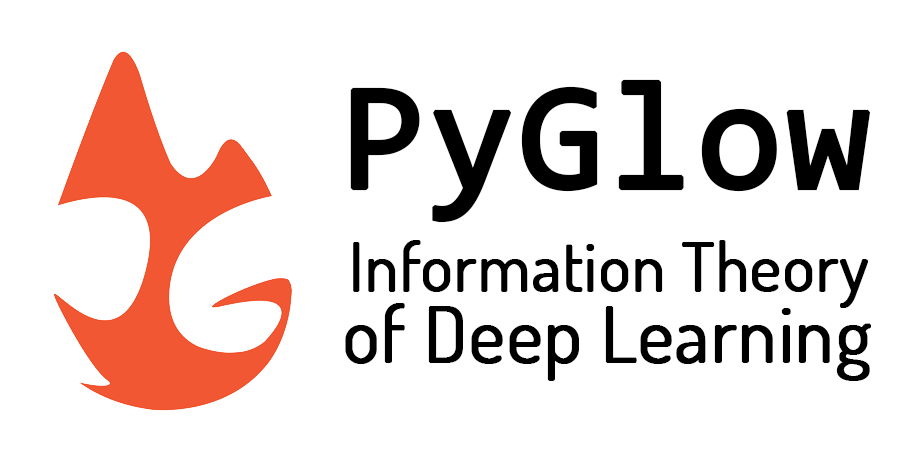# Welcome to PyGlow’s documentation!¶

PyGlow is a Python package which attempts to implement Keras like API struture on PyTorch backend. It provides functionalities which supports information theoretic methods in deep learning. These methods are relevant for understanding neural network dynamics in information plane. The package is equipped with a number of state-of-the-art algorithms for estimating and calculating various kinds of information theoretic measures. The package also provides intensive support for information bottleneck based methods in deep learning.

## What’s new with PyGlow ?¶

PyGlow provide support for the following features of the ‘IB theory of Deep Learning’:

• Functionalities for experimental IB-based DNN analysis
• Test IB-based DNN performance bounds
• Flexible API structure to test theoretical ideas and hypothesis related to IB-theory or information theory in general
• IB-based training paradigms for DNN
• Flexible internal pipeline which allows for the implementation of custom user-defined dependence criterions or IB-based loss functions

## Example¶

Let’s see a code snippet which tracks the input-hidden-label segment (called dynamics segment) for each batch of each epoch and calculates the HSIC criterion between each hidden layer, input and label of a dynamics segment which is for each layer of each batch of each epoch.

 ``` 1 2 3 4 5 6 7 8 9 10 11 12 13 14 15 16 17 18 19 20 21 22 23 24 25 26 27 28 29 30 31 32 33 34 35 36 37``` ```# importing PyGlow modules import glow from glow.layers import Dense, Dropout, Conv2d, Flatten from glow.datasets import mnist, cifar10 from glow.models import IBSequential from glow.information_bottleneck.estimator import HSIC # hyperparameter batch_size = 64 num_workers = 3 validation_split = 0.2 num_epochs = 2 # load the dataset train_loader, val_loader, test_loader = mnist.load_data( batch_size=batch_size, num_workers=num_workers, validation_split=validation_split ) model = IBSequential(input_shape=(1, 28, 28), gpu=True, track_dynamics=True, save_dynamics=True) model.add(Conv2d(filters=16, kernel_size=3, stride=1, padding=1, activation='relu')) model.add(Flatten()) model.add(Dropout(0.4)) model.add(Dense(500, activation='relu')) model.add(Dropout(0.4)) model.add(Dense(200, activation='relu')) model.add(Dropout(0.2)) model.add(Dense(10, activation='softmax')) # compile the model model.compile(optimizer='SGD', loss='cross_entropy', metrics=['accuracy']) # attach evaluator - Tracks the dynamics and calculate coordinates model.attach_evaluator(HSIC(kernel='gaussian', gpu=True, sigma=5)) # train the model along with calculating dynamics model.fit_generator(train_loader, val_loader, num_epochs) ```

That’s it ! you can now extract the evaluated dynamics from the model. For complete code checkout this notebook.

You can find more example on either at Jupyter Notebooks section or related notebooks in github repo at examples.

And yes the code structure looks exactly like Keras ! PyGlow closely follows the API structure of Keras and provides with an ‘easy to implement’ pipeline so that you can really work on your ideas rather than debugging ;)

Now that you have seen the exciting implications of PyGlow and with how much clean interface you can implement information theoretic methods for your own model, just head over to install it on your local machine at Getting Started and get everything set up to really open the black box of deep learning !